﻿ 舰载导弹发射筒指向精度研究
 舰船科学技术2019, Vol. 41Issue (4): 142-147PDF

Research on pointing accuracy of a ship-based missile launch canister
WANG Yong, ZHAO Xi-lei, LI Xiang-yu
The 713 Research Institute of CSIC, Zhengzhou 450015, China
Abstract: Pointing accuracy is an important index to the precision of missile launch canister . Providing the initial angle when a missile is launched, pointing accuracy is a vital factor deciding whether a missile will hit its target precisely . This research paper decides the factors that influence the precision of missile launch canister by analysing the overall composition of a ship-based oblique fixed missile launch canister and puts forward a reliable and systematic research method based on the comprehensive analysis of RMS and Maximum's error and the comparison of data . To some degree, this research method decreases the influence of phase angle of various factors that influence the precision of missile launch canister and reflects the pointing accuracy relatively truthfully, offering instructive function and application value to the overall design of missile launch canister.
Key words: pointing accuracy     missile launch canister     RMS     maximum     phase angle
0 引　言

1 影响导弹发射筒指向精度因素分析 1.1 导弹发射筒结构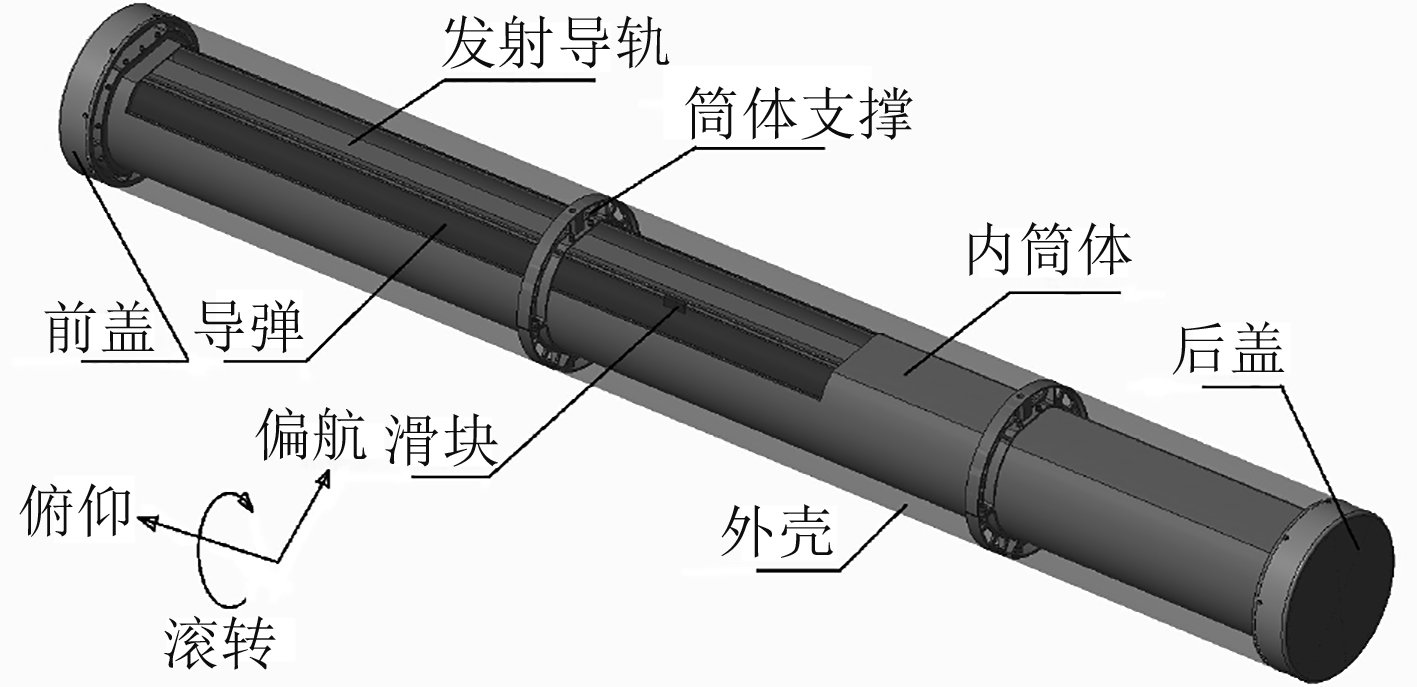图 1 导弹发射筒结构示意图 Fig. 1 The structure schematic diagram of a missile launch canister
1.2 导弹发射筒指向精度的评估和分配

1.3 影响导弹发射筒指向精度的因素分析

1.3.1 导弹的影响图 2 某舰载导弹结构示意图 Fig. 2 The structure schematic diagram of a ship-based missile
1.3.1.1 导弹滑块与导弹中心线安装精度的影响

 ${{\rm{\beta }}_{{{D_{1}}}}} = {\rm{arctan}}\left( {{{\rm{\delta }}_{{{PX_{1}}}}}/{{{L}}_{{{HK_{1}}}}}} \right){\text{。}}$ (1)

 ${{\rm{\gamma }}_{{{D_{1}}}}} = {\rm{arctan}}\left( {{{\rm{\delta }}_{{{PX_{2}}}}}/{{{L}}_{{{HK_{2}}}}}} \right){\text{。}}$ (2)

 ${{\rm{\alpha }}_{{{D_{1}}}}} = {\rm{arctan}}\left( {{{\rm{\delta }}_{{{DC_{1}}}}}/{{{L}}_{{{HK_{1}}}}}} \right){\text{。}}$ (3)
1.3.1.2 导弹滑块与发射导轨配合精度的影响

 ${{\rm{\alpha }}_{{{D_{2}}}}} = {\rm{arctan}}\left( {{{\rm{\delta }}_{{{JX_{1}}}}}/{{{L}}_{{{DG_{1}}}}}} \right){\text{。}}$ (4)

 ${{\rm{\beta }}_{{{D_{2}}}}} = {\rm{arctan}}\left( {{{\rm{\delta }}_{{{JX_{2}}}}}/{{{L}}_{{{DG_{1}}}}}} \right){\text{。}}$ (5)
1.3.2 发射筒的影响 1.3.2.1 发射导轨的影响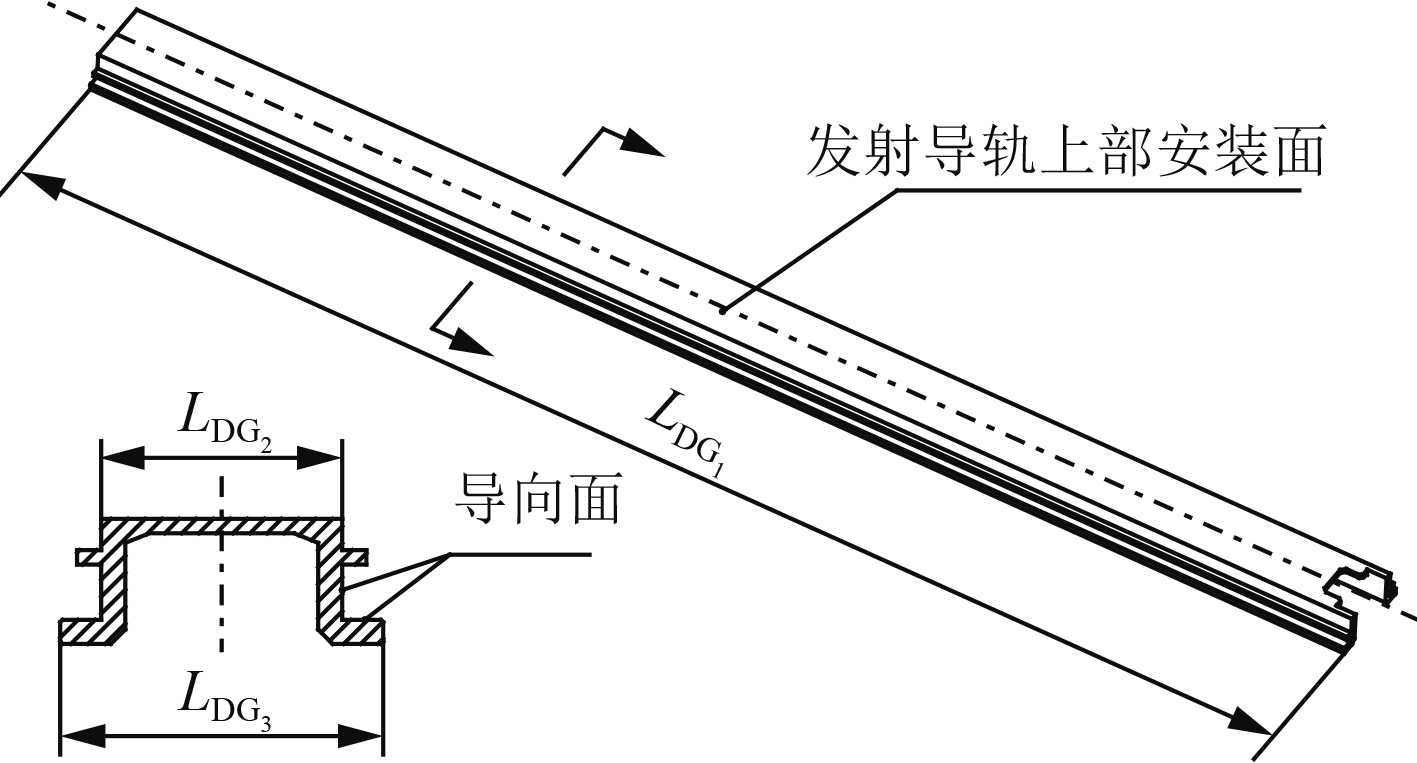图 3 发射导轨结构示意图 Fig. 3 The structure schematic diagram of launching guide

1）发射导轨上部安装面加工精度的影响

 ${{\rm{\beta }}_{{{T_{1}}}}} = {\rm{arctan}}\left( {{{\rm{\delta }}_{{{ZX_{1}}}}}/{{{L}}_{{{DG_{1}}}}}} \right){\text{。}}$ (6)

 ${{\rm{\gamma }}_{{{T_{1}}}}} = {\rm{arctan}}\left( {{{\rm{\delta }}_{{{QX_{1}}}}}/{{{L}}_{{{DG_{2}}}}}} \right){\text{。}}$ (7)

2）发射导轨与导弹滑块配合面加工精度的影响

 ${{\rm{\beta }}_{{{T_{2}}}}} = {\rm{arctan}}\left( {{{\rm{\delta }}_{{{PX_{3}}}}}/{{{L}}_{{{DG_{1}}}}}} \right){\text{。}}$ (8)

 ${{\rm{\gamma }}_{{{T_{2}}}}} = {\rm{arctan}}\left( {{{\rm{\delta }}_{{{PX_{4}}}}}/{{{L}}_{{{DG_{3}}}}}} \right){\text{。}}$ (9)

 ${{\rm{\alpha }}_{{{T_{1}}}}} = {\rm{arctan}}\left( {{{\rm{\delta }}_{{{DC_{2}}}}}/{{{L}}_{{{DG_{1}}}}}} \right){\text{。}}$ (10)

3）发射导轨安装精度的影响

 ${{\rm{\alpha }}_{{{T_{2}}}}} = {\rm{arctan}}\left( {{{\rm{\delta }}_{{{AZ_{1}}}}}/{{{L}}_{{{DG_{1}}}}}} \right){\text{。}}$ (11)
1.3.2.2 发射筒内筒体安装面制造精度的影响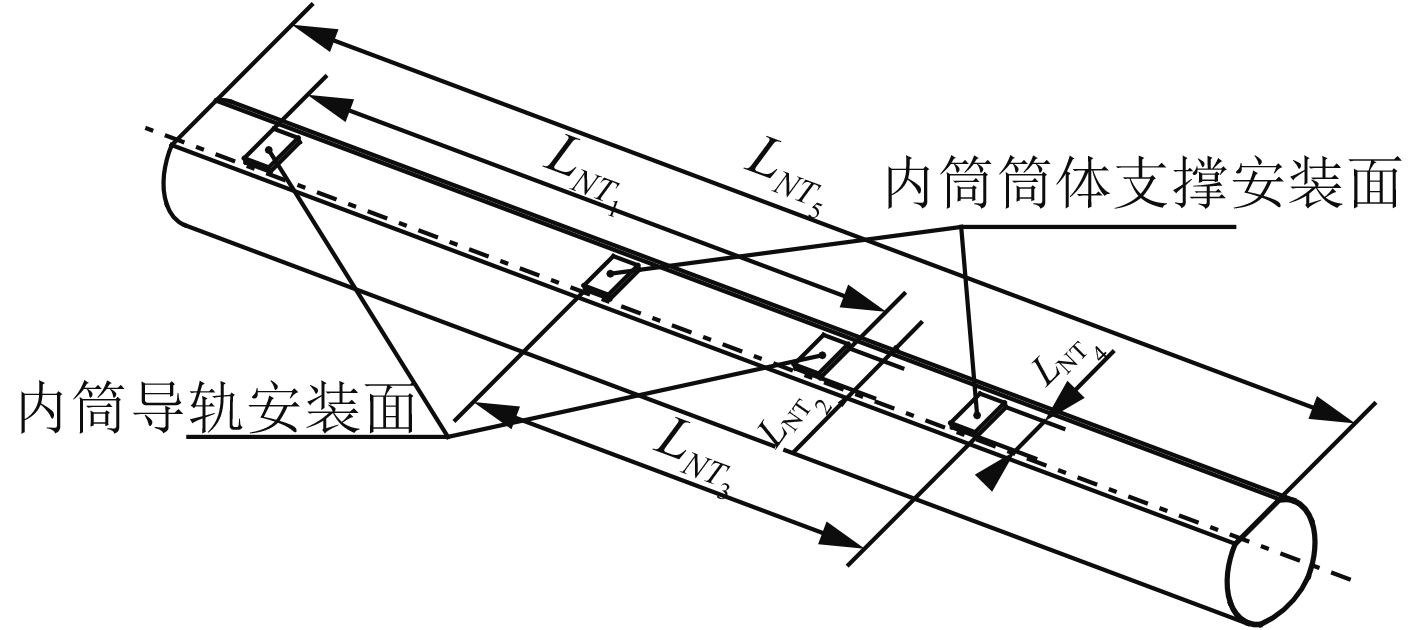图 4 内筒体结构示意图 Fig. 4 The structure schematic diagram of inner cylinder

1）发射筒内筒导轨安装面制造精度的影响

 ${{\rm{\beta }}_{{{T_{3}}}}} = {\rm{arctan}}\left( {{{\rm{\delta }}_{{{PX_{5}}}}}/{{{L}}_{{{NT_{1}}}}}} \right){\text{。}}$ (12)

 ${{\rm{\gamma }}_{{{T_{3}}}}} = {\rm{arctan}}\left( {{{\rm{\delta }}_{{{PX_{6}}}}}/{{{L}}_{{{NT_{2}}}}}} \right){\text{。}}$ (13)

2）发射筒内筒筒体支撑安装面制造精度的影响

 ${{\rm{\beta }}_{{{T_{4}}}}} = {\rm{arctan}}\left( {{{\rm{\delta }}_{{{PX_{7}}}}}/{{{L}}_{{{NT_{3}}}}}} \right){\text{。}}$ (14)

 ${{\rm{\gamma }}_{{{T_{4}}}}} = {\rm{arctan}}\left( {{{\rm{\delta }}_{{{PX_{8}}}}}/{{{L}}_{{{NT_{4}}}}}} \right){\text{。}}$ (15)
1.3.2.3 筒体支撑的影响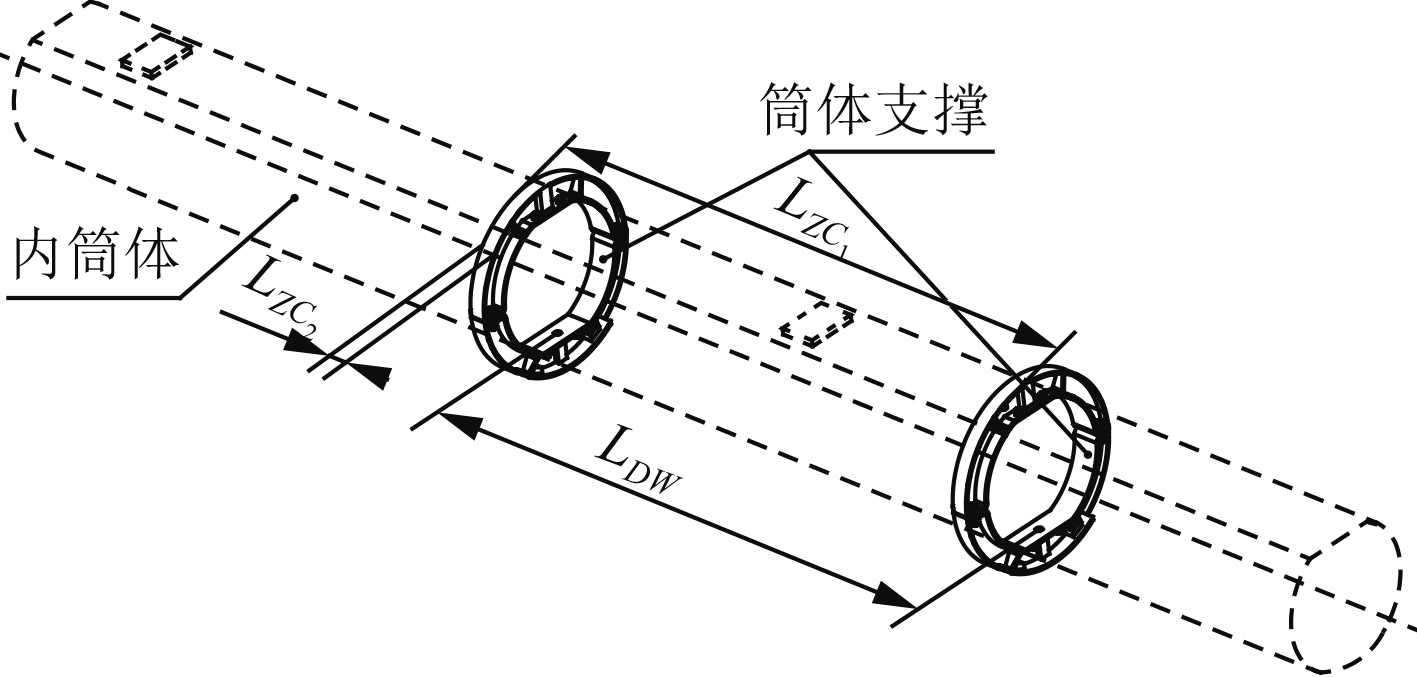图 5 筒体支撑结构示意图 Fig. 5 The structure schematic diagram of cylinder support

1）筒体支撑制造精度的影响

 ${{\rm{\beta }}_{{{T_{5}}}}} = {\rm{arctan}}\left( {{{\rm{\delta }}_{{{PX_{9}}}}}/{{{L}}_{{{ZC_{1}}}}}} \right){\text{，}}$ (16)

 ${{\rm{\gamma }}_{{{T_{5}}}}} = {\rm{arctan}}\left( {{{\rm{\delta }}_{{{PX_{10}}}}}/{{{L}}_{{{ZC_{2}}}}}} \right){\text{。}}$ (17)

2）筒体支撑与内筒安装精度的影响

 ${{\rm{\alpha }}_{{{T_{3}}}}} = {\rm{arctan}}\left( {{{\rm{\delta }}_{{{AZ_{2}}}}}/{{{L}}_{{{NT_{3}}}}}} \right){\text{。}}$ (18)
1.3.2.4 发射筒与发射支架安装精度的影响

 ${{\rm{\alpha }}_{{{T_{4}}}}} = {\rm{arctan}}\left( {{{\rm{\delta }}_{{{JX_{3}}}}}/{{{L}}_{{{DW}}}}} \right){\text{。}}$ (19)
1.3.2.5 发射筒结构刚度的影响

 ${{\rm{\alpha }}_{{\rm{T}}{\text{结构刚度}}}} = {\rm{arctan}}({{\rm{\Delta }}_{{\text{横向}}}}/{{{L}}_{{{NT_{5}}}}}){\text{，}}$ (20)
 ${{\rm{\beta }}_{{\rm{T}}{\text{结构刚度}}}} = {\rm{arctan}}({{\rm{\Delta }}_{{\text{法向}}}}/{{{L}}_{{{NT_{5}}}}}){\text{。}}$ (21)
1.3.2.6 导弹发射筒各因素引起的指向误差汇总表 1 指向误差汇总 Tab.1 Pointing error summary

2 导弹发射筒指向误差统计方法 2.1 统计方法

1）导弹发射筒的偏航误差α偏航误差统计方法如下：

 $\begin{split} {\alpha _{\text{偏航误差}}} =& {\alpha _D} + {\alpha _T} = {\alpha _D} + \left( {{\alpha _{{\rm{T}}{\text{静态}}}} + {\alpha _{{\rm{T}}{\text{动态}}}}} \right)=\\ & \sqrt {\displaystyle\sum\limits_{{{k}} = 1}^{{n}} {{\alpha _{{\rm{Dk}}}}^2} } + \left( {\sqrt {\displaystyle\sum\limits_{{{j}} = 1}^{{m}} {{\alpha _{{\rm{Tj}}}}^2} } + {\alpha _{{\rm{T}}{\text{结构刚度}}}}}\!\! \right){\text{。}} \end{split}$ (22)

2）导弹发射筒的俯仰误差β俯仰误差统计方法如下：

 $\begin{split} {\beta _{\text{俯仰误差}}} &= {\beta _D} + {\beta _T} = {\beta _D} + \left( {{\beta _{{\rm{T}}{\text{静态}}}} + {\beta _{{\rm{T}}{\text{动态}}}}} \right)=\\ & \sqrt {\displaystyle\sum\limits_{{{k}} = 1}^{{n}} {{\beta _{{\rm{Dk}}}}^2} } + \left( {\sqrt {\displaystyle\sum\limits_{{{j}} = 1}^{{m}} {{\beta _{{\rm{Tj}}}}^2} } + {\beta _{{\rm{T}}{\text{结构刚度}}}}} \right){\text{。}} \end{split}$ (23)

3）导弹发射筒的滚转误差γ滚转误差统计方法如下：

 ${\gamma _{\text{滚转误差}}} = {\gamma _{\rm{D}}} + {\gamma _{\rm{T}}} = \sqrt {\sum\limits_{{{k}} = 1}^{{n}} {{\gamma _{{\rm{Dk}}}}^2} } + \sqrt {\sum\limits_{{{j}} = 1}^{{m}} {{\gamma _{{\rm{Tj}}}}^2} } {\text{。}}$ (24)

2.2 统计结果和数据分析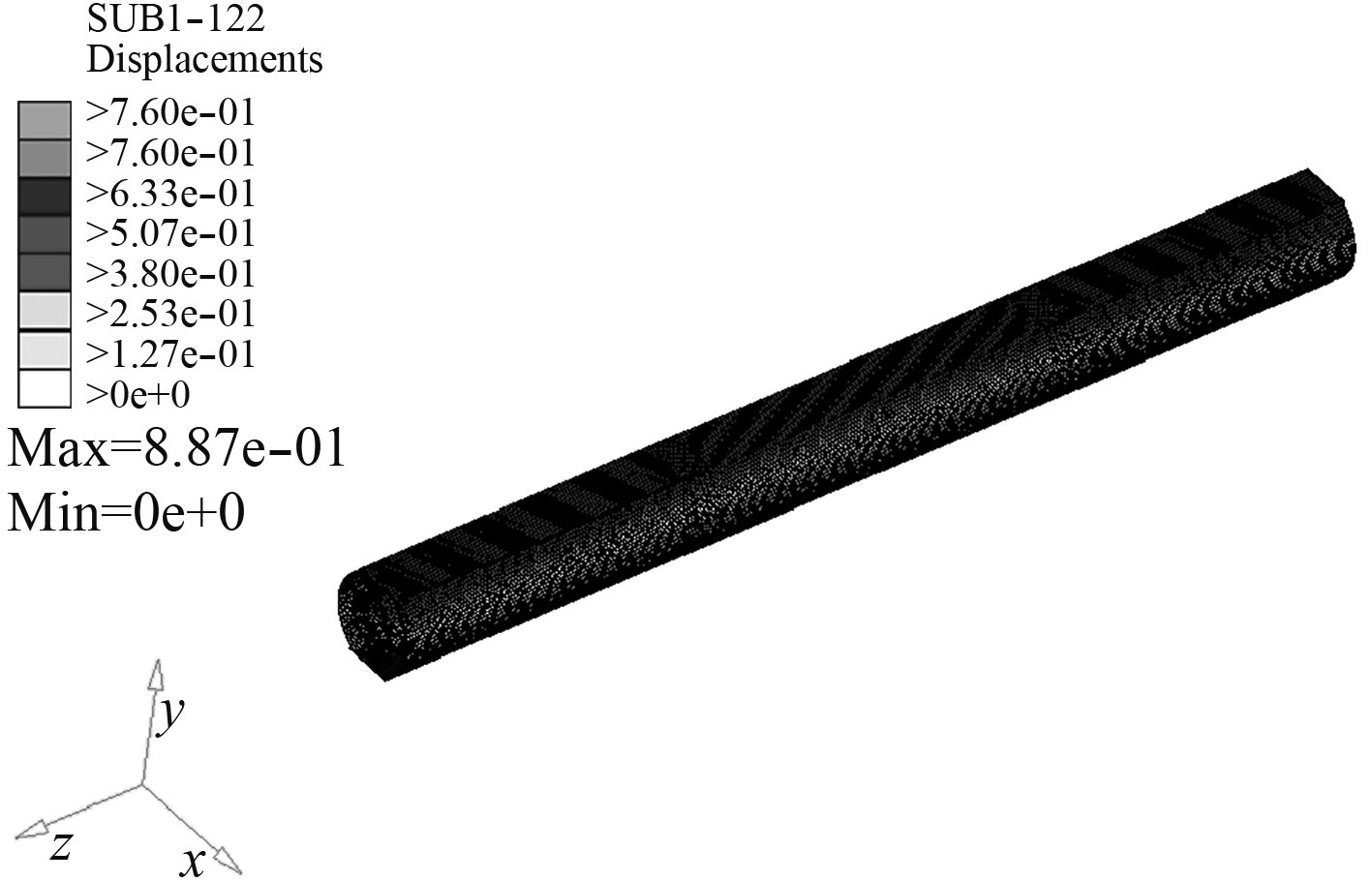图 6 发射筒有限元仿真结果 Fig. 6 Finite element simulation results of launch canister表 2 指向误差数据对比表 Tab.2 Pointing error data contrast table

3 提高导弹发射筒指向精度的措施 3.1 提高各关键部件的加工和安装精度

3.2 合理的进行结构设计

3.3 减少误差源

4 结　语

  姚昌仁, 张波. 火箭导弹发射装置设计[M]. 北京: 北京理工大学出版社, 1998.  徐挺, 李斐, 王霞. 指导系统精度分析及误差分配方法[J]. 四川兵工学报, 2014, 35(3): 18-20. XU Ting, LI Fei, WANG Xia. Accuracy analysis and error distribution of guidance system[J]. Journal of Sichuan Ordance, 2014, 35(3): 18-20. DOI:10.11809/scbgxb2014.03.005  王晨晨, 胡祥涛. 光电稳定平台指向误差建模与分析[J]. 新型工业化, 2014, 4(2): 86-90. WANG Chen-chen, HU Xiang-tao. The modeling and analysis of opto-electronic stabilized platform pointing accuracy[J]. The Journal of New Industrialization, 2014, 4(2): 86-90.  吴秉贤, 严世泽, 龚龙兴. 火箭发射装置结构分析[M]. 北京: 国防工业出版社, 1988.  叶期传, 徐辅仁, 王新华. 用概率统计法计算齿轮机构的回差[J]. 机电设备, 2003(1): 16-19. YE Qi-chuan, XU Fu-ren, WANG Xin-hua. Calculating the return stagnant angle of the gearing by use of probability statistic[J]. Mechanical and Electrical Equipment(Marine), 2003(1): 16-19. DOI:10.3969/j.issn.1005-8354.2003.01.003  张家华. 某型跟踪雷达传动误差及回差测量方法[J]. 舰船电子对抗, 2002, 25(2): 40-41. ZHANG Jia-hua. Measurement method of transmission error and return stagnant for a tracking radar[J]. Shipboard Electronic Countermeasure, 2002, 25(2): 40-41. DOI:10.3969/j.issn.1673-9167.2002.02.012  孙新学, 李文武, 荣茜, 等. 提高机械传动精度的方法和措施[J]. 机床与液压, 2004(3): 166-170. SUN Xin-xue, LI Wen-wu, RONG Qian, et al. Methods and measures to improve the precision of mechanical transmission[J]. Machine Tool & Hydraulics, 2004(3): 166-170.